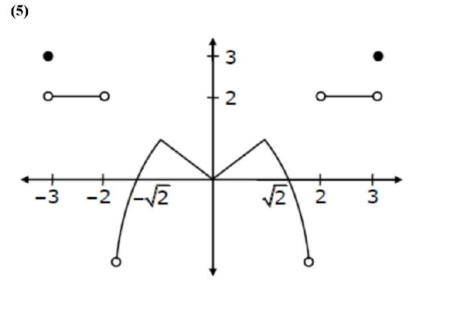# A function f is defined on [-3,3] asQuestion:

A function $f$ is defined on $[-3,3]$ as

$f(x)=\left\{\begin{array}{cc}\min \left\{|x|, 2-x^{2}\right\}, & -2 \leq x \leq 2 \\ {[|x|]} & , 2<|x| \leq 3\end{array}\right.$

where $[\mathrm{x}]$ denotes the greatest integer $\leq \mathrm{x}$. The number of points, where $\mathrm{f}$ is not differentiable in $(-3,3)$ is

Solution:Points of non-differentiability in $(-3,3)$ are at $x=-2,-1,0,1,2$.

i.e. 5 points.